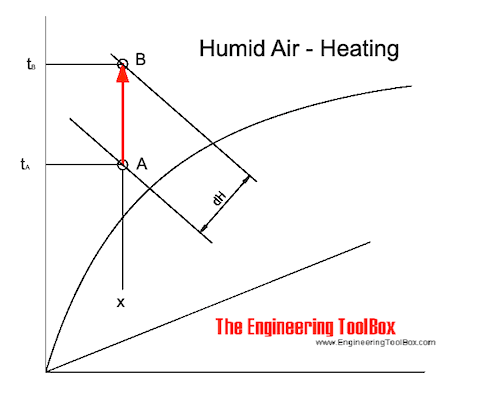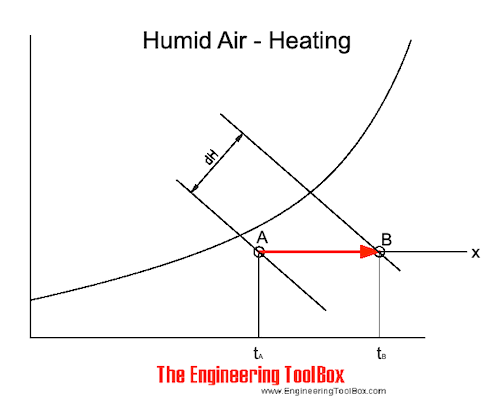Engineering ToolBox - Resources, Tools and Basic Information for Engineering and Design of Technical Applications!

# Humid Air - Heating

## Enthalpy change and temperature rise when heating humid air without adding moisture.

The process of sensible heating of air - heating without adding moisture - can be visualized in the Mollier diagram as:Sensible heating of air changes the state of the air from A to B along the constant specific humidity - x - line. The supplied heat - dH - can be estimated as indicated in the diagram above.

The heating process can also be visualized in the psychrometric chartNote! - when sensible heating of air - the specific moisture remains constant - the relative humidity is decreased.

### Calculating Enthalpy

The enthalpy of moist air can be calculated as:

h = cpa t + x [cpw t + hwe]                            (1)

where

h = specific enthalpy of moist air (kJ/kg)

cpa = 1.01 - specific heat capacity of air at constant pressure (kJ/kgoC, kWs/kgK)

t = air temperature (oC)

x = humidity ratio (kg/kg)

cpw = 1.84 - specific heat capacity of water vapor at constant pressure (kJ/kg.oC, kWs/kg.K)

hwe = 2502 - evaporation heat of water at 0oC (kJ/kg)

(1) can be modified to:

h = 1.01 (kJ/kg.oC) t + x [1.84 (kJ/kg.oC) t + 2502 (kJ/kg)]                                 (1b)

### The Enthalpy Difference

The enthalpy difference when heating air without adding moisture can be calculated as:

dhA-B = cpa tB + x [cpw tB + hwe] - cpa tA + x [cpw tA + hwe]

= cpa(tB - tA) + x cpw (tB - tA)                                                (2)

#### Example - Enthalpy Change when Heating Air

The specific humidity of air at 25oC and relative moisture 50% is 0.0115 kg/kg - check the Mollier diagram. The change in enthalpy when heating the air to 35oC can be calculated as:

dhA-B = (1.01 kJ/kgoC)(35oC - 25oC) + (0.0115 kg/kg) (1.84 kJ/kgoC) (35oC - 25oC)

= (10.1 kJ/kg) + (0.2 kJ/kg)

= 10.3 (kJ/kg)

Note! - the contribution from the water vapor is relatively small and can for practical purposes often be neglected. (2) can then be modified to:

dhA-B = cpa( tB - tA)                                 (2b)

### Increase in Temperature when Heating Air

If heat is added to humid air the increase in air temperature can be calculated by modifying (2b) to:

tB - tA = dhA-B / cpa                                  (2c)

#### Example - Heating Air and Temperature Rise

If 10.1 kJ is added to 1 kg air the temperature rise can be calculated as:

tB - tA = (10.1 kJ/kg) / (1.01 kJ/kgoC)

= 10 (oC)

### Heat Flow in a Heating Coil

The total heat flow rate through a heating coil can be calculated as:

q = m (hB - hA)                                     (3)

where

q = heat flow rate (kJ/s, kW)

m = mass flow rate of air (kg/s)

The total heat flow can also be expressed as:

qs = L ρ (hB - hA)                                     (3a)

where

L = air flow rate (m3/s)

ρ = density of air (kg/m3)

Note! The density of air varies with temperature. At 0oC the density is 1.293 kg/m3. At 80oC the density is 1.0 kg/m3.

It's common to express sensible heat flow rate as:

q = m cpa (tB - tA)                                  (3b)

or alternatively:

q = L ρ cpa (tB - tA)                                   (3c)

### Heating Coil Effectiveness

For a limited heating coil surface the average surface temperature will always be higher than the outlet air temperature. The effectiveness of a heating coil can be expressed as:

μ = (tB - tA) / (tHC - tA)                                   (4)

where

μ = heating coil effectiveness

tHC = mean surface temperature of the heating coil (oC)

### Example - Heating Air

1 m3/s of air at 15oC and relative humidity 60% (A) is heated to 30oC (B). The surface temperature of the heating coil is 80oC. The density of air at 20oC is 1.205 kg/m3.

From the Mollier diagram the enthalpy in (A) is 31 kJ/kg and in (B) 46 kJ/kg.

The heating coil effectiveness can be calculated as:

μ = (30oC - 15oC) / (80oC - 15oC)

= 0.23

The heat flow can be calculated as:

q = (1 m3/s) (1.205 kg/m3) ((46 kJ/kg) - (31 kJ/kg))

= 18 (kJ/s, kW)

As an alternative, as one of the most common methods:

q = (1 m3/s) (1.205 kg/m3) (1.01 kJ/kg.oC) (30oC - 15oC)

= 18.3 (kJ/s, kW)

Note! Due to the inaccuracy when working with diagrams there is a small difference between the total heat flow and the sum of the latent and sensible heat. In general - this inaccuracy is within acceptable limits.

## Related Topics

• ### Air Psychrometrics

Moist and humid air - psychrometric charts, Mollier diagrams, air-condition temperatures and absolute and relative humidity and moisture content.

## Related Documents

• ### Air - Drying Force

The drying force of air depends on the air moisture holding capacity and the water surface to air evaporation capacity.
• ### Air - Heating, Cooling, Mixing, Humidifying or Dehumidifying Processes

Basic air changing state processes - heating, cooling, mixing, humidifying and dehumidifying by adding steam or water - psychometric diagrams and the Mollier charts.
• ### Air - Humidifying with Steam, SI units

Using steam to humidify air.
• ### Air - Humidity Measurement from Dry and Wet Bulb Temperature

Relative humidity in moist air can estimated by measuring the dry and wet bulb temperature.
• ### Air - Maximum Moisture Carrying Capacity

Maximum water content in humid air vs. temperature.
• ### Air - Specific Heat vs. Temperature at Constant Pressure

Online calculator with figures and tables showing specific heat (Cp and Cv) of dry air vs. temperature and pressure. SI and imperial units.
• ### Air - Thermal Conductivity vs. Temperature and Pressure

Online calculator with figures and tables showing air thermal conductivity vs. temperature and pressure. SI and imperial units.
• ### Air - Thermophysical Properties

Thermal properties of air at different temperatures - density, viscosity, critical temperature and pressure, triple point, enthalpi and entropi, thermal conductivity and diffusivity and more.
• ### Air Cooling of Storage Roooms

Heat removed from storage rooms with cooled air.
• ### Air Heating Systems

Air heating buildings - heat supply vs. air flow and temperature.
• ### Cooling and Heating Equations

Latent and sensible cooling and heating equations - imperial units.
• ### Cooling Load - Latent and Sensible Heat

Latent and sensible cooling loads to consider when designing HVAC systems.
• ### Dry Air - Thermodynamic and Physical Properties

Thermodynamic properties of dry air - specific heat, ratio of specific heats, dynamic viscosity, thermal conductivity, Prandtl number, density and kinematic viscosity at temperatures ranging 175 - 1900 K.
• ### Great Sensible Heat Factor - GSHF

The Great Sensible Heat Factor is the ratio sensible to total heat in a cooling coil.
• ### Heat Recovery

Ventilation and heat-recovery calculations, sensible and latent heat - online calculators - imperial units.
• ### Heat Recovery Efficiency

Classification of heat recovery efficiencies - temperature efficiency, moisture efficiency and enthalpy efficiency - online heat exchanger efficiency calculator.
• ### Latent Heat Flow

Latent heat is the heat when supplied to or removed from air results in a change in moisture content - the temperature of the air is not changed.
• ### Moist Air - Cooling and Dehumidifying

Cooling and dehumidifying processes of moist and humid air - sensible and latent cooling.
• ### Moist Air - Enthalpy

Sensible and latent heat of moist air.
• ### Removing Heat with Air

Calculating heat removed with air by measuring the wet bulb temperature.
• ### Steam Heating Air

Calculate steam heated air systems.

## Engineering ToolBox - SketchUp Extension - Online 3D modeling!

Add standard and customized parametric components - like flange beams, lumbers, piping, stairs and more - to your Sketchup model with the Engineering ToolBox - SketchUp Extension - enabled for use with older versions of the amazing SketchUp Make and the newer "up to date" SketchUp Pro . Add the Engineering ToolBox extension to your SketchUp Make/Pro from the Extension Warehouse !

We don't collect information from our users. More about

## Citation

• The Engineering ToolBox (2004). Humid Air - Heating. [online] Available at: https://www.engineeringtoolbox.com/heating-humid-air-d_693.html [Accessed Day Month Year].

Modify the access date according your visit.

9.19.12